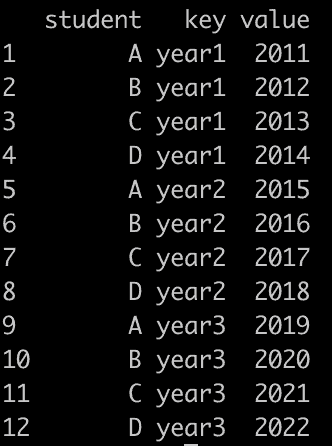# How to Use the gather() Function in R

The gather() function in R is “used to gather a key-value pair across multiple columns”.

## Syntax

``gather(data, key value)``

## Parameters

1. data: It is the name of the data frame.
2. key: It is the name of the key column to create.
3. value: It is the name of the value column to create

## Example 1: Gather Values From Two Columns

``````library(tidyr)

data_wide <- data.frame(
id = c(1, 2, 3),
variable1 = c(5, 6, 7),
variable2 = c(10, 11, 12)
)

# Convert the data frame from wide to long format
data_long <- gather(data_wide, key = "variable", value = "value", -id)

# Print the long data frame
print(data_long)
``````

Output

``````   id  variable   value
1  1   variable1    5
2  2   variable1    6
3  3   variable1    7
4  1   variable2   10
5  2   variable2   11
6  3   variable2   12
``````

## Example 2: Gather Values From More than Two Columns

To gather values from more than two columns in R, you can use the “gather()” function from the tidyr package.

``````library(tidyr)

# create data frame
df <- data.frame(
student = c("A", "B", "C", "D"),
year1 = c(2011, 2012, 2013, 2014),
year2 = c(2015, 2016, 2017, 2018),
year3 = c(2019, 2020, 2021, 2022)
)

# Gather the values from the year1, year2, and year3 columns.
df_gathered <- gather(df, key, value, year1, year2, year3)

# Print the data frame.
print(df_gathered)
``````

OutputAs mentioned earlier, the gather() function has been superseded by the pivot_longer() function in the tidyr package.

It is recommended to use pivot_longer() for new projects, as it offers a more consistent and flexible interface for reshaping data.

That’s it.